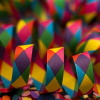#### You may also like### Repetitiously

Can you express every recurring decimal as a fraction?

# Tiny Nines

### Why do this problem

There are fascinating patterns to be found in recurring decimals. This problem explores the relationship between fraction and decimal representations. It's a great opportunity to practise converting fractions to decimals with and without a calculator.

### Possible approach

This problem could be explored alongside Repetitiously

Show students the video in the problem, or ask them to find the decimal representations of $\frac19$, $\frac1{99}$, and $\frac1{999}$ for themselves. Invite them to predict what $\frac1{9999}$ will be as a decimal.

Challenge students to convince themselves, and convince you, that the decimal representations really do go on forever. They may do this by performing a division calculation by hand and considering the remainders, or converting the recurring decimals back into a fraction (as demonstrated in Repetitiously). Then they could explore other related fractions such as those suggested in the problem:

• $\frac13$, $\frac{1}{33}$, $\frac{1}{333}$...

• $\frac1{11}$, $\frac1{111}$, $\frac1{1111}$...

• $\frac{23}{99}$, $\frac{37}{99}$, $\frac{52}{99}$, $\frac{n}{99}$

### Key questions

How can a fraction be turned into a decimal representation?
Without using a calculator?

If you know that $\frac19=0.\dot{1}$, how can you work out $\frac13$ as a decimal?
If you know that $\frac1{99}=0.0\dot{1}$, how can you work out $\frac1{11}$ as a decimal?

### Possible support

If they haven't already done so, students could start by exploring Terminating or Not.

### Possible extension

Challenge students to prove that the patterns they have noticed will continue.